• 华南理工大学SE编译原理实验4 - 中间代码生成
• 编译原理实验报告——生成中间代码，从文件读入表达式，输出其四元式的结果序列
• 如果不生成中间代码而是直接生成机器语言或者汇编语言形式的目标代码，优点是编译时间短，缺点是目标代码执行效率和质量都比较低，移植性差。 1.2 表示形式 逆波兰式（后缀式）、三地址码（三元式、四元式）、...


1.概述
1.1 定义
源程序的一种内部表示，不依赖目标机的结构，易于机械生成目标代码的中间表示。如果不生成中间代码而是直接生成机器语言或者汇编语言形式的目标代码，优点是编译时间短，缺点是目标代码执行效率和质量都比较低，移植性差。
1.2 表示形式
逆波兰式（后缀式）、三地址码（三元式、四元式）、抽象语法树、有向无环图。
1.3 地位
如下所示：2.逆波兰式
2.1 定义
把运算量（操作数）写在前面，把运算符写在后面，因此又称为后缀表示法
2.2 案例
如下所示：
表达式语句逆波兰式a+bab+(a+b)*cab+c*a+b*cabc*+a=b*c+b*dabc*bd*+=

3.三元地址
3.1 定义
指每条代码包含一个运算和三个地址，两个地址用于存放运算对象，一个地址用于存放运算结果。其一般形式为
x=y op z。
3.2 四元式
3.2.1 定义
一个四元式具有四个域的记录结构，表示为：（op，arg1，arg2，result）。op为运算符；result存放运算结果；arg为运算对象，如果为空，则使用空格，留出位置。
3.2.2 案例
例：将 a=b*c+b*d用四元式形式
写成三地址码的赋值语句形式如下：
1) t1 = b*c
2) t2 = b*d
3) t3 =t1+t2
4) a = t3
写成四元形式如下：
1) (*,b,c,t1)
2) (*,b,d,t2)
3) (+,t1,t2,t3)
4) (=,t3, ,a)
3.3 三元式
3.3.1 定义
一个三元式具有三个域的记录结构，表示为：（op，arg1，arg2）。
3.3.2 案例
例：将 a=b*c+b*d用三元形式
(1) (*,b,c)
(2) (*,b,d)
(3) (+,(1),(2))
(4) (=,(3),a)
3.4 抽象语法树
3.4.1 定义
抽象语法树（Abstract Syntax Code，AST）是语法树的一种简化形式，是源程序的抽象语法结构的树状表示，树的每个节点都表示源代码中的一种结构。
3.5 有向无环图
3.5.1 定义
有向无环图（Directed Acyclic Graph，简称DAG）对表达式中的每个子表达式都有一个结点，内部结点表示运算符，它的孩子代表运算分量。DAG中代表公共子表达式的结点只出现一次，具有多个父结点。


展开全文• 编译课程设计——中间代码生成部分，输入为二元式文档，输出为四元式，并且有数据结构存储，visual c++编写
• 编译原理中间代码生成器实现C++编译原理中间代码生成器实现C++
• 了解目标代码生成阶段在编译处理过程中的功能和作用 •了解常用的三种目标代码形式及其优缺点 •了解虚拟机及其指令系统 •深入理解并掌握有中间代码向目标代码转换的过程和原理
• 文章目录基础DAG三地址代码问题声明语句的翻译表达式和赋值语句的翻译控制流翻译布尔表达式的翻译switch 语句的翻译过程调用的翻译回填 基础 DAG 语法树是一种图形化的中间表示。但语法树，公共子表达式每出现一次...


文章目录
基础DAG三地址代码
问题声明语句的翻译表达式和赋值语句的翻译控制流翻译布尔表达式的翻译switch 语句的翻译过程调用的翻译回填

基础
DAG
语法树是一种图形化的中间表示。但语法树中，公共子表达式每出现一次，就有一个对应的子树，产生了大量冗余， 因此定义了另一种中间表示：有向无环图(Directed Acyclic Graph, DAG) 有向无环图的构造与抽象语法树类似。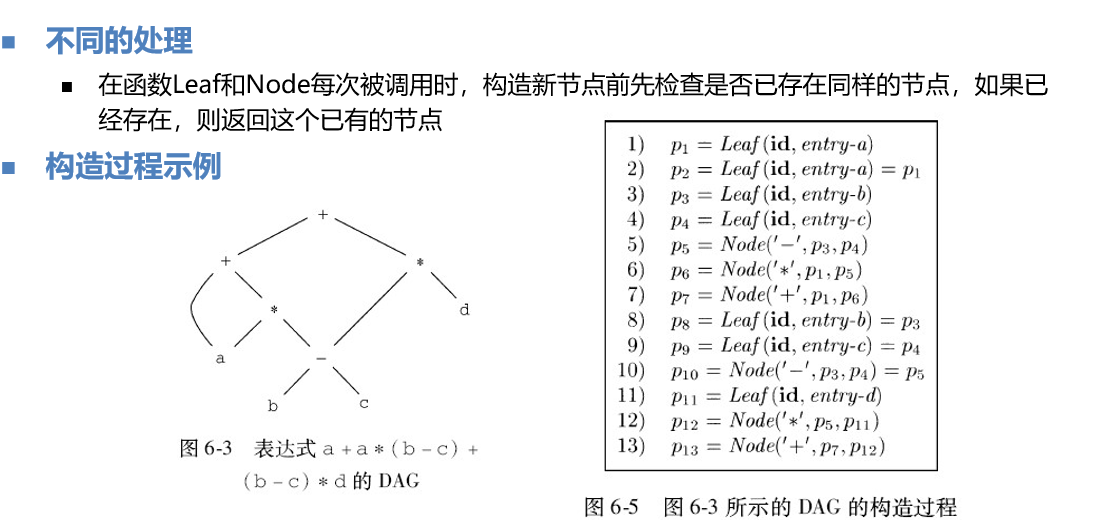三地址代码
一般包括一个运算符和之多三个运算分量(地址)，所以叫做三地址代码。这里的地址包括：变量、常量、临时变量。
指令集合
运算/赋值指令：x=y op z ，x = op y复制指令：x=y无条件转移指令：goto L条件转移指令：if x goto L ，ifFalse x goto L条件转移指令：if x relop y goto L过程调用/返回 p(x1, x2, …, xn) • param x1 //设置参数 • param x2 • … • param xn • call p, n //调用子过程p，n为参数个数带下标的复制指令：x=y[i] x[i]=y • 注意：i表示离开数组位置第i个字节，而不是数组的第i个元素地址/指针赋值指令： • x=&y x=*y *x=y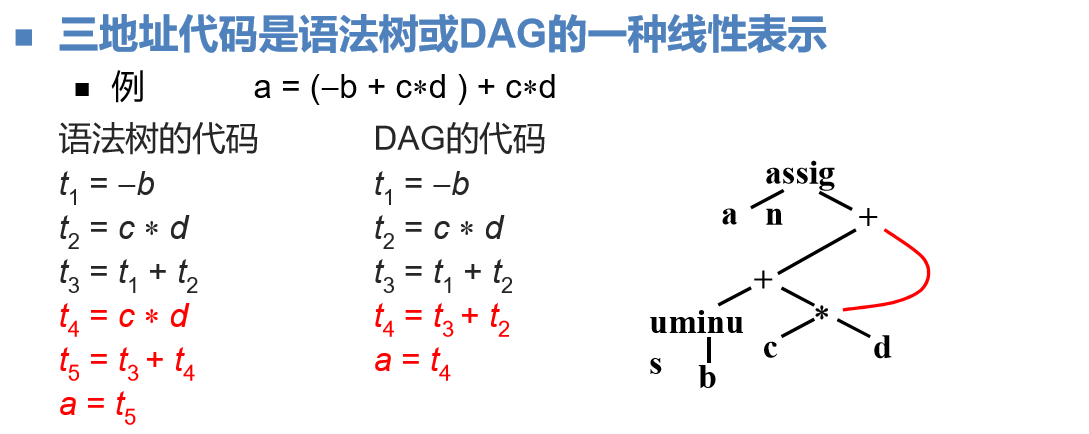三地址代码的四元式表示 格式（字段）： op arg1 arg2 result 如：+ x y z 对于有些指令，一些参数是空缺的，如 param x1 null null [if goto] x null L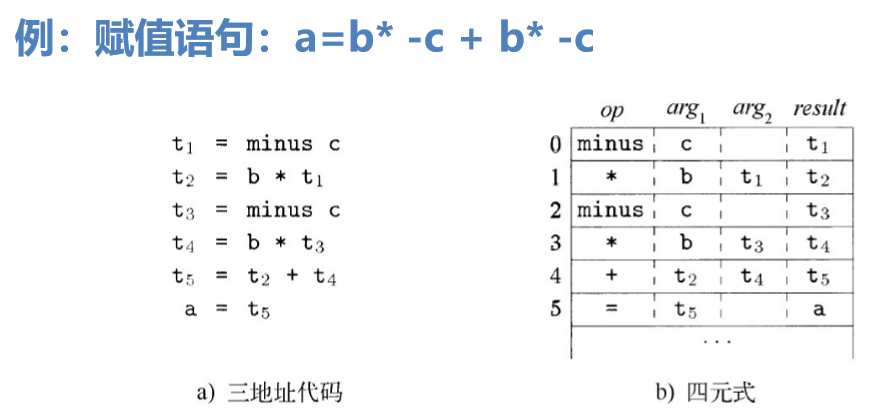三地址代码的三元式表示 op arg1 arg2 使用三元式的位置来引用三元式的运算结果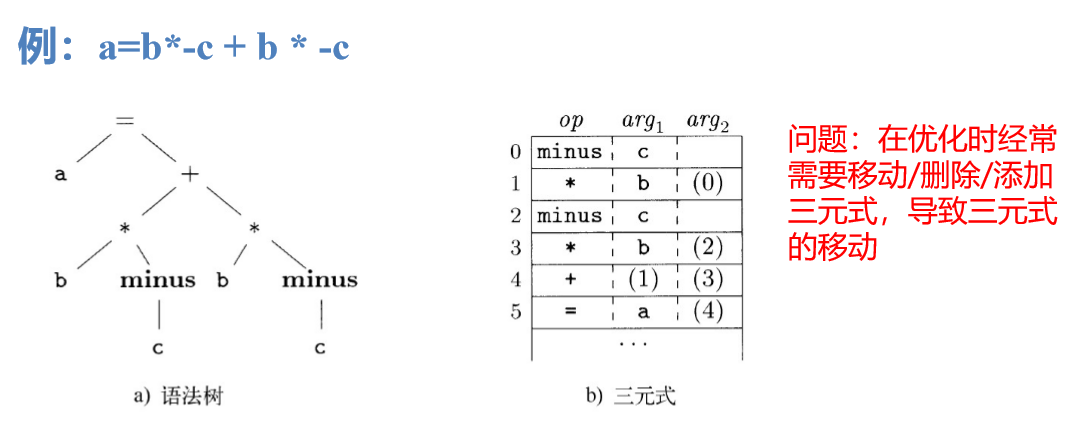三地址代码的间接三元式表示 包含了一个指向三元式的指针的列表  我们可以对这个列表进行操作，完成优化功能；操作时不 需要修改三元式中的参数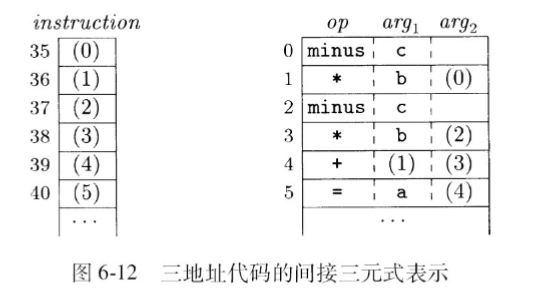静态单赋值SAA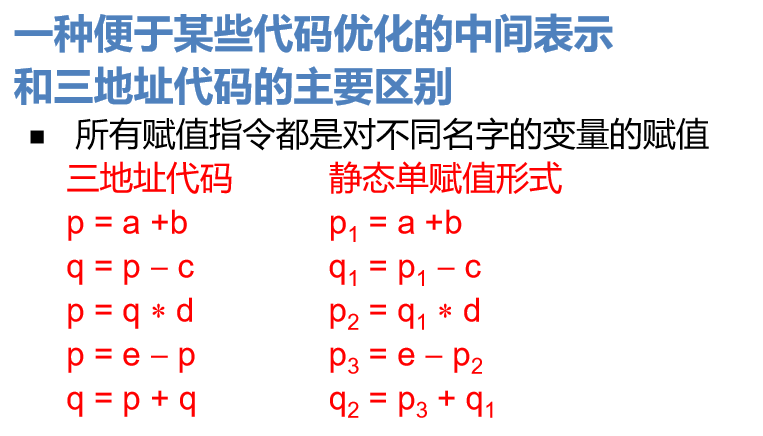问题
声明语句的翻译
PPT 24-54
表达式和赋值语句的翻译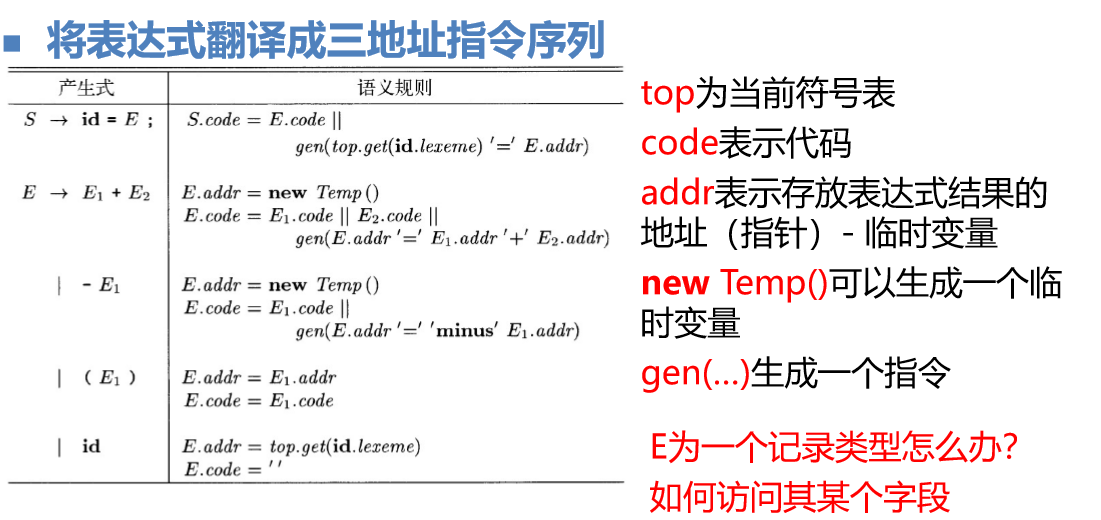数组引用生成代码的翻译方案
非终结符号L的三个综合属性
L.addr指示一个临时变量，计算数组引用的偏移量 ij * wjL.array是一个指向数组名字对应的符号表条目的指针， L.array.base为该数组的基地址L.type是L生成的子数组的类型，对于任何数组类型t，其宽度由 t.width给出，t.elem给出其数组元素的类型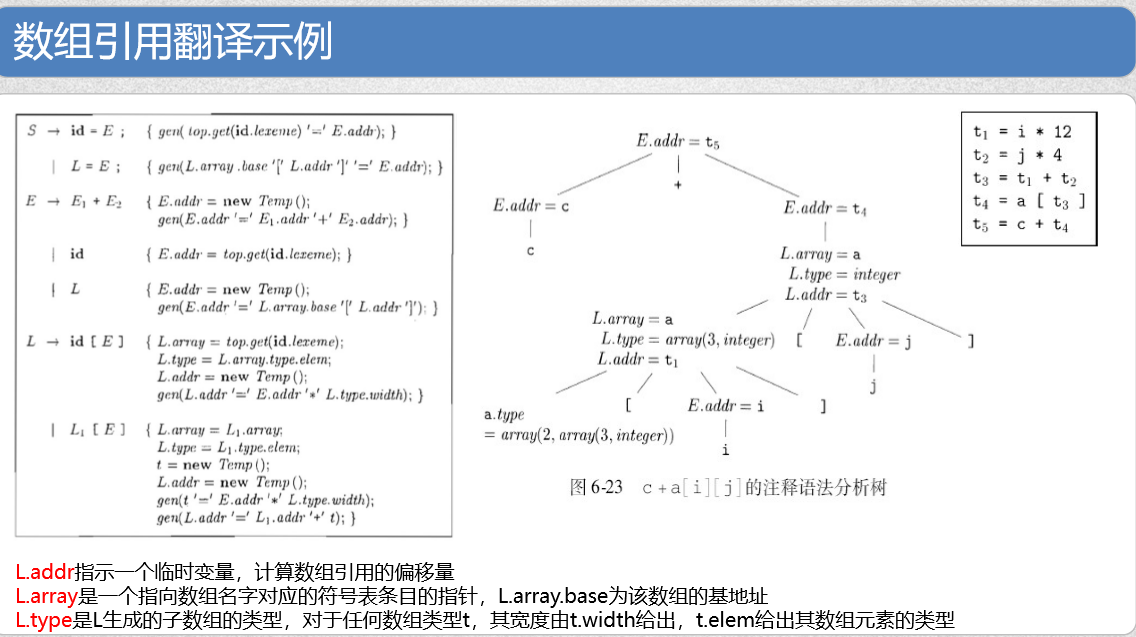控制流翻译
文法：B表示布尔表达式，S代表语句 S → if (B) S1 ; S → if (B) S1 else S2 ;S → while (B) S1 . 代码的布局见下图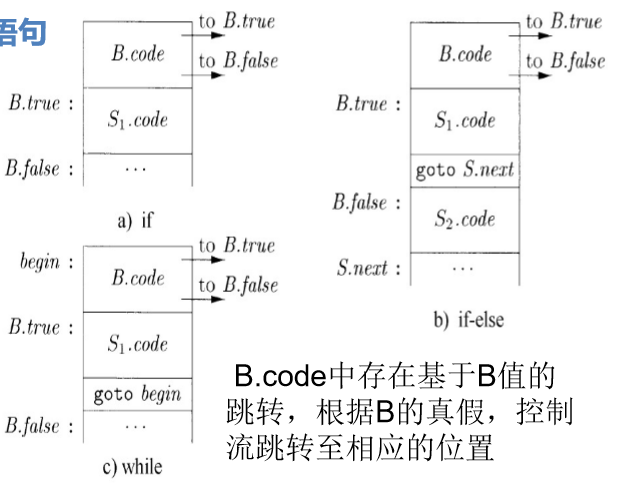继承属性
B.true：B为真的跳转目标B.false：B为假的跳转目标S.next：S执行完毕时的跳转目标
例：

S

⟶

i

f

B

t

h

e

n

S

1

S\longrightarrow if\ B\ then\ S_1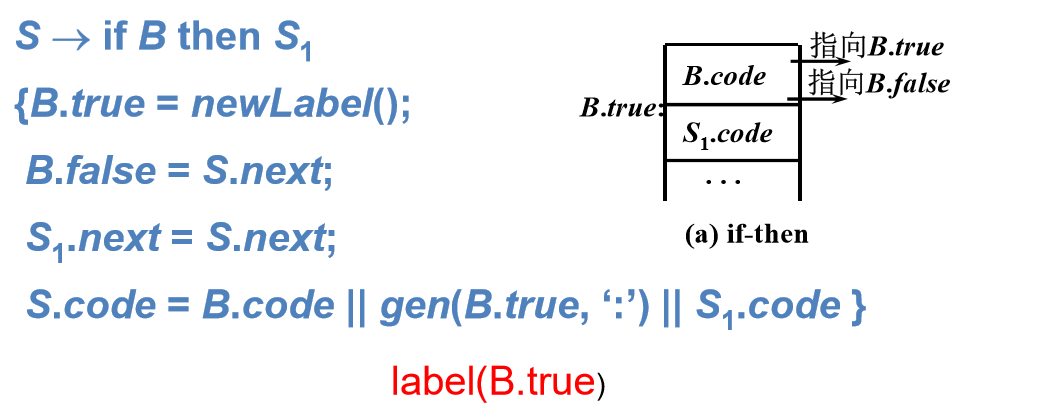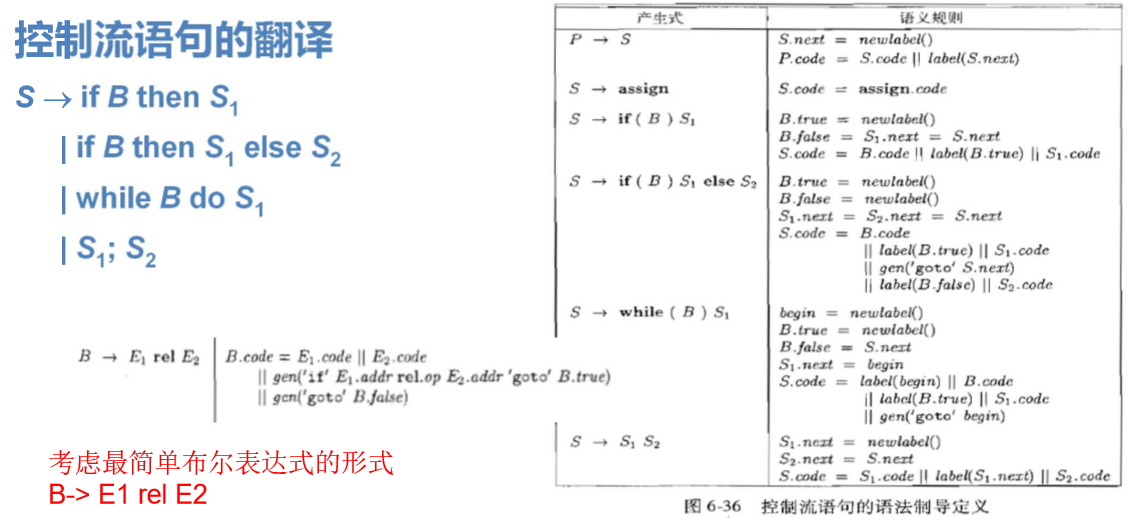布尔表达式的翻译
当布尔表达式的计算用于跳转分治
布尔表达式翻译的主要语义动作是跳转，而跳转的标签主要根据短路规则来确定。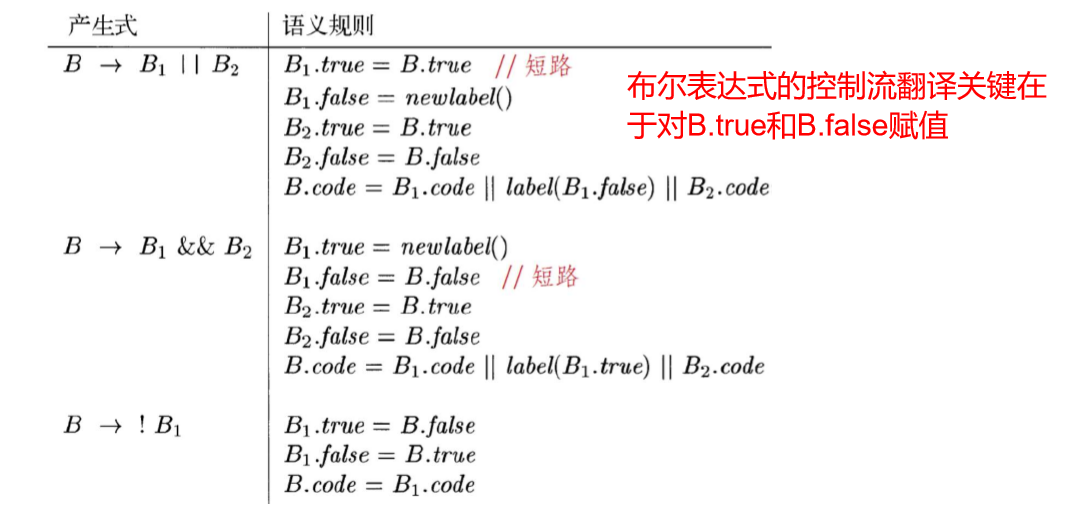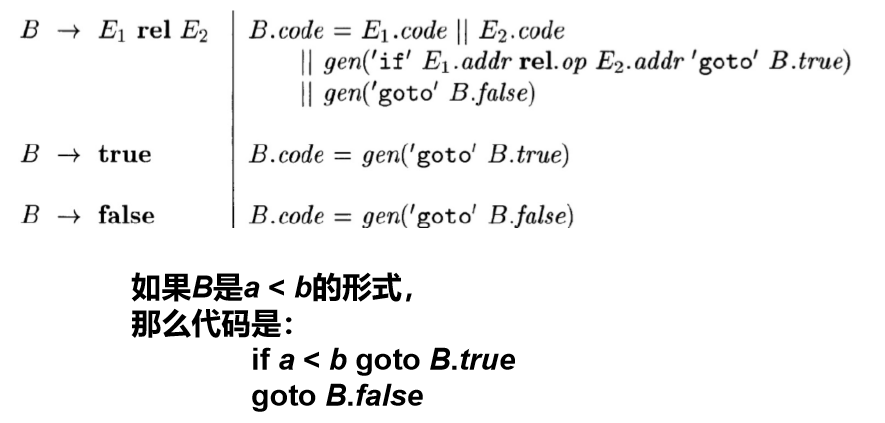当布尔表达式的计算用于赋值 采用临时变量的方法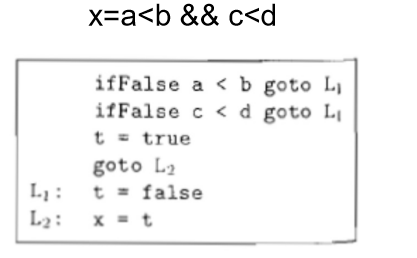switch 语句的翻译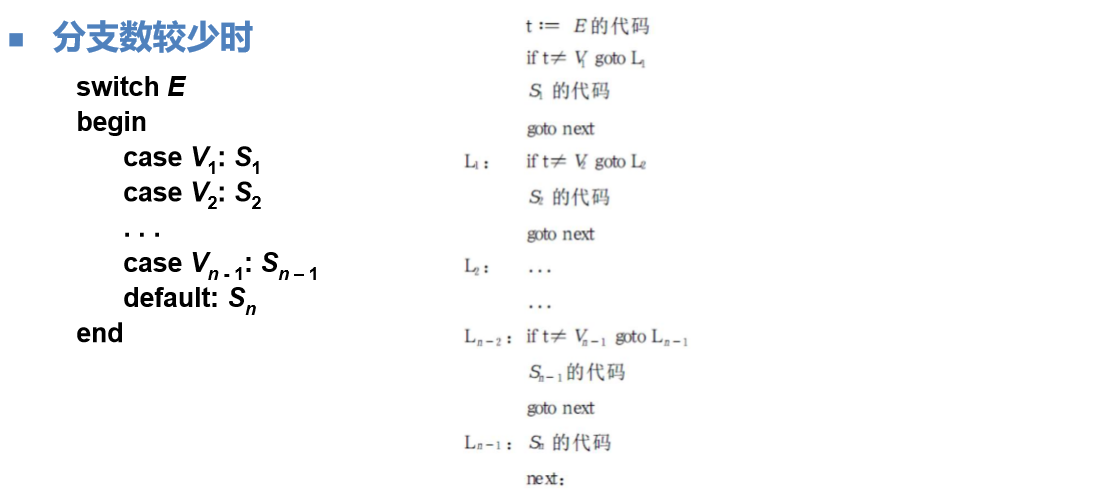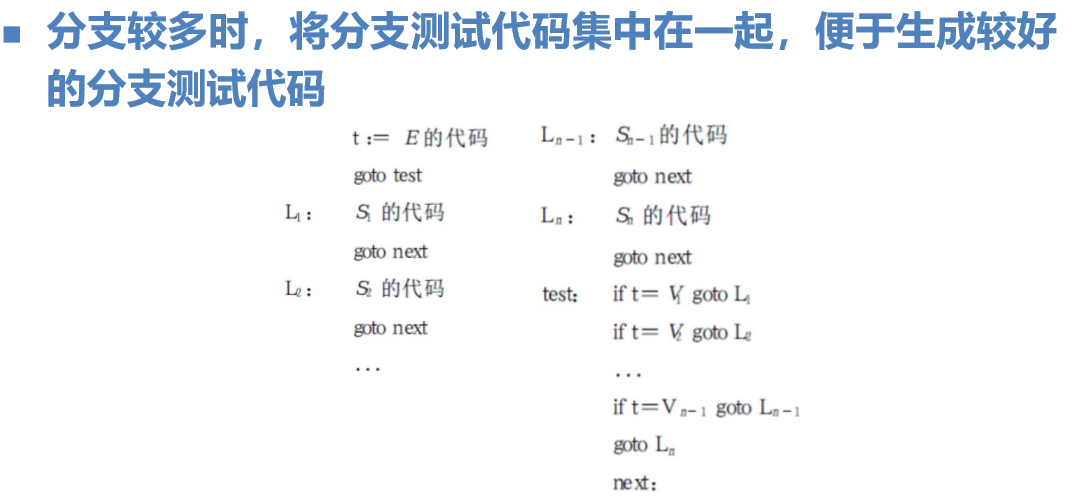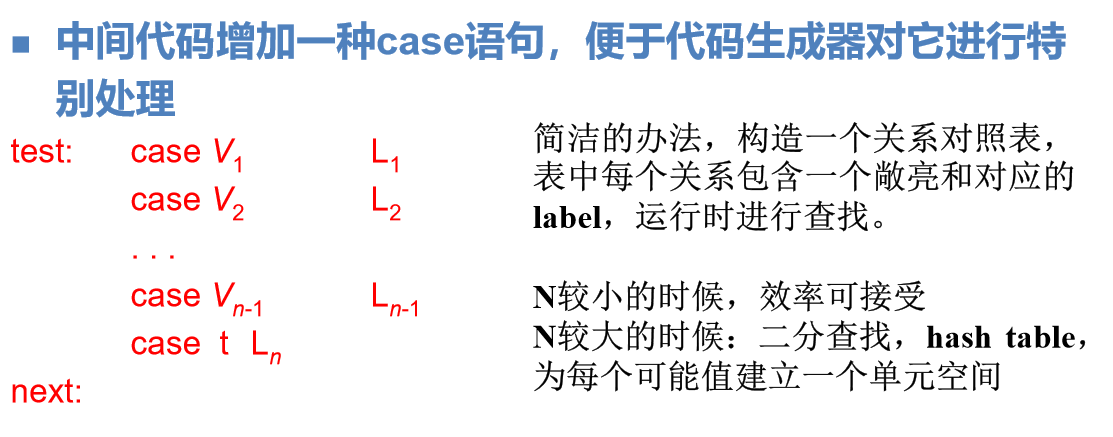过程调用的翻译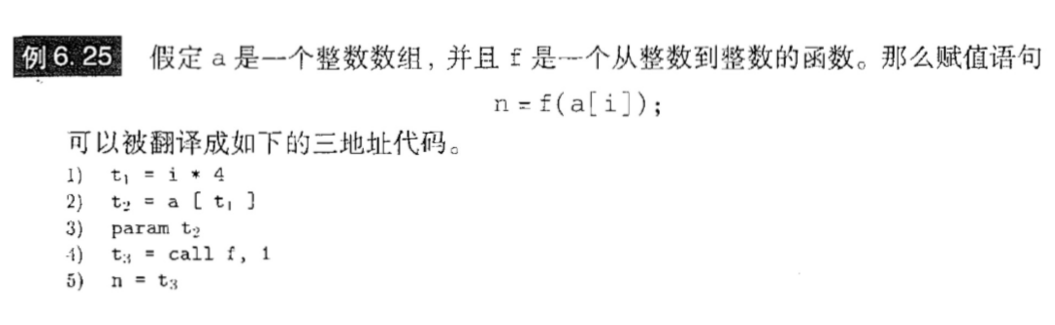回填
布尔表达式用于语句的控制流时，它总是在取值true时和取值false 时分别跳转到某个位置
引入两个综合属性
truelist: 包含跳转指令（位置）的列表，这些指令在取值true时执行falselist：包含跳转指令（位置）的列表，这些指令在取值false时执行  辅助函数
Makelist(i)：创建一个只包含i的列表Merge(p1,p2)：将p1和p2指向的列表合并Backpatch(p,i)：将i作为目标标号插入到p所指列表中的各指令中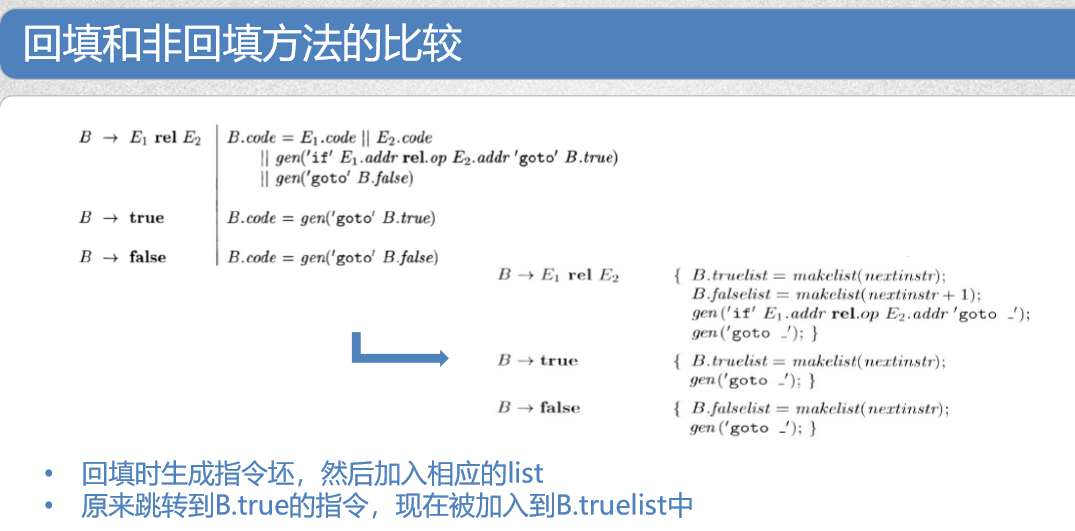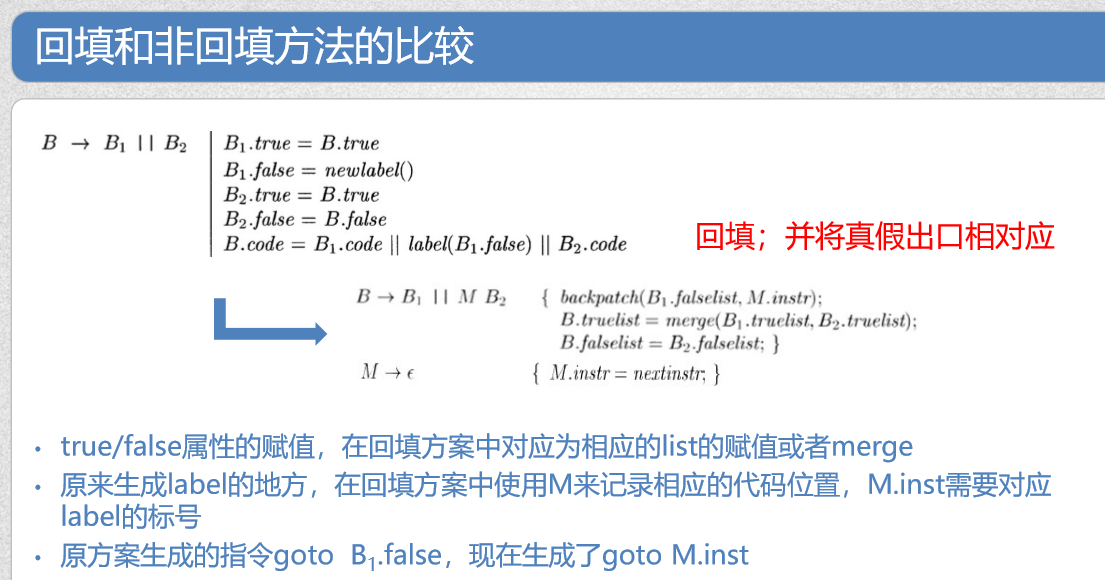控制转移语句的回填 M的作用就是用M.instr记录下一个指令的位置 • N的作用是生成goto指令坯，N.nextlist只包含这个指令 的位置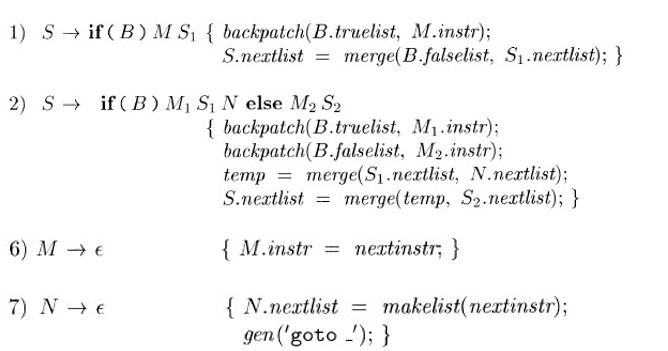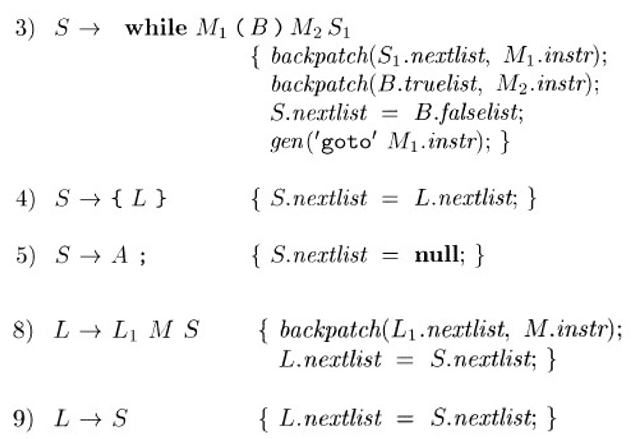展开全文三地址代码 布尔表达式的翻译
• 如果不生成中间代码而是直接生成机器语言或者汇编语言形式的目标代码，优点是编译时间短，缺点是目标代码执行效率和质量都比较低，移植性差。 为什么不直接翻译成机器码呢，而多此一举生成中间代码再转换？（代码的...
中间代码定义

源程序的一种内部表示，不依赖目标机的结构，易于机械生成目标代码的中间表示。 如果不生成中间代码而是直接生成机器语言或者汇编语言形式的目标代码，优点是编译时间短，缺点是目标代码执行效率和质量都比较低，移植性差。

为什么不直接翻译成机器码呢，而多此一举生成中间代码再转换？（代码的鲁棒性）

是为了提高编译器的可移植性，因为不同的cpu的指令集是不一样的，假如直接翻译成机器代码，那么当你换了一块cpu之后，可能你的编译器就完全不能运行了，所以为了鲁棒性，将代码先翻译成中间代码，然后在能在多个不同的cpu上做出相应的改变并且运行了。使用中间代码的好处:
1）一是便于编译器程序的开发和移植（鲁棒性） 2）二是代码进行优化处理
常见的中间代码表示形式

逆波兰式（后缀式）、三地址码（三元式、四元式）、抽象语法树、有向无环图。

逆波兰式 运算量（操作数）写在前面，把运算符写在后面，因此又称为后缀表示法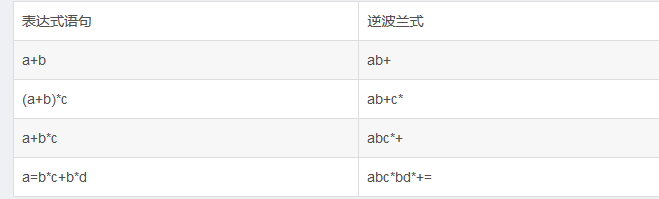三地址码——最常用的中间代码形式是三地址码，它的实现形式常采用四元式形式。 指每条代码包含一个运算和三个地址，两个地址用于存放运算对象，一个地址用于存放运算结果。其一般形式为x=y op z。 四元式 一个四元式具有四个域的记录结构，表示为：（op，arg1，arg2，result）。op为运算符；result存放运算结果；arg为运算对象，如果为空，则使用空格，留出位置。 四元式与三元式的唯一区别： 将由序号所表示的运算结果改为：用(临时)变量来表示。 此改变使得四元式的运算结果与其在四元式序列中的位置无关.为代码的优化提供了极大方便，因为这样可以删除或移动四元式而不会影响运算结果.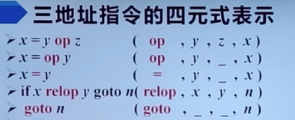例：将 a=b*c+b*d用三元形式
(1) (*,b,c)
(2) (*,b,d)
(3) (+,(1),(2))
(4) (=,(3),a)

例：将 a=b*c+b*d用四元式形式
写成三地址码的赋值语句形式如下：
1) t1 = b*c
2) t2 = b*d
3) t3 =t1+t2
4) a = t3
写成四元形式如下：
1) (*,b,c,t1)
2) (*,b,d,t2)
3) (+,t1,t2,t3)
4) (=,t3, ,a)


抽象语法树 抽象语法树（Abstract SyntaxCode，AST）是语法树的一种简化形式，是源程序的抽象语法结构的树状表示，树的每个节点都表示源代码中的一种结构。

有向无环图 有向无环图（Directed Acyclic Graph，简称DAG）对表达式中的每个子表达式都有一个结点，内部结点表示运算符，它的孩子代表运算分量。DAG中代表公共子表达式的结点只出现一次，具有多个父结点。

一个例子

声明语句的翻译之变量的声明 一个变量的声明应该由两部分来完成：类型的定义和变量的声明 类型定义：为编译器提供存储空间大小的信息 变量声明：为变量分配存储空间 组合数据的类型定义和变量声明：定义与声明在一起，定义与声明分离.

引用于介篇文章
展开全文• c＋＋实现的中间代码生成语法分析的基础上，对所要分析的文档输出四元式形式。代码能有运行有注释。有使用说明。自己编译原理已通过的作业。
• 编译程序锁使用的中间代码有多种形式。常见的有逆波兰记号，三元式，四元式，和树形表示。四元式是一种普遍采用的中间代码形式，很类似于三地址指令，有时把这类中间表示称为“三地址代码”，这种表示可以看作是一种...
预备知识
源语言->中间代码->目标语言  中间代码（Intermediate Representation或者IR）:复杂性介于源程序语言和机器语言的一种表示形式。  编译程序锁使用的中间代码有多种形式。常见的有逆波兰记号，三元式，四元式，和树形表示。四元式是一种普遍采用的中间代码形式，很类似于三地址指令，有时把这类中间表示称为“三地址代码”，这种表示可以看作是一种虚拟三地址机的通用汇编码，每条”指令“包含操作符和三个地址，两个是为运算对象的，一个是为结果的。  基本表达式的翻译模式：  (1)  Exp:Exp ASSIGNOP Exp  |Exp AND Exp  |Exp OR Exp  |Exp PLUS Exp  |Exp MINUS Exp  |Exp STAR Exp  |Exp DIV Exp  |ID  |INTEGER  |FLOAT  ;  分析：  （1）要给每一个语法树结点增加一个place属性，这个place属性的值根据以下规则计算。  1）如果EXP->ID,不需要为ID建立临时变量，place的值就等于变量ID的名字；  2）如果EXP->INTEGER|FLOAT，即推出常数，不需要为常数建立临时变量，place的值就等于常数值。  3）E->E1=E2,E1->ID
emit($1);printf("=");emit($3);
step1：输出ID;  step2:输出=  step3:输出E2.place
4）i+1;E->E1+E2，newtemp，建立一个临时变量，E.place=E1.place+E2.place,
$$>t=newtemp();//创建临时变量 emit($$);//输出创建E.place
printf("=");//输出=
emit($1);//输出E1.place printf("+");//输出+ emit($3);//输出E2.place
也就是说，在语法树的叶子结点存在两种place，一种是ID，即变量名，一种是整数或者浮点数，这两种place值都和临时变量无关，不需要建立临时变量的操作。  place的值对应到四元式中：  1）常数  2）源程序中变量名ID  3）为生成四元式而建立的临时变量名。  方法一：变量名采用t加上数字的形式，为所有的临时变量名创建一个符号表，记其在符号表中的位置，这个位置是一个在整数v，从0开始，输出时，输出’t’+v,就可以输出t0,t1这种形式，而且有效地避免了变量名的重复。  方法二：直接将place设置成整数类型，再设置一个标记数组used[]，用了一个整数i，就在标记数组中将used[i]=-1,表示ti已经被用过了。这样一来，创建一个临时变量，相当于设置place的值，然后更改used[]数组的值。
place应该是一个Union，有3种可能值。  1）int i(对应INTEGER)  2）char* (对应变量名ID)  3)float (对应FLOAT)  4)int t;对应临时变量编号  为了能识别place的值是4种中哪一种，再为语法树结点增添一个ptag,1为int,2为float，3为char,4为临时变量编号。
union
{
int i;
char* s;
float f;
}place;
int tag;//标志位

int main()
{
place.s="ID";
tag=1;
if(tag==1)
printf("%s",place);
}

除了EXP->ID,EXP->常数，每一条基本表达式规则要有一个输出四元式的操作，emit。
代码更改：  由于用union导致了内存的错乱（具体什么原因，过后还需要研究），因此将union去掉，4种类型都设置为语法树结点的成员，这样一来可能浪费了空间，但是能保证代码的正确性。
一、【实验目的】
通过在词法分析，语法分析和语义分析程序的基础上，将C—源代码翻译成中间代码，认识中间代码的表示形式和生成中间代码的原理和技巧，掌握对简单赋值语句的翻译过程，从而达到对编译器的编译原理有更深的理解，提高代码能力和代码修养。
二、【实验任务】
在词法分析，语法分析和语义分析程序的基础上，将C—源代码翻译成中间代码，中间代码的表示采用四元式，本实验中只对基本表达式进行翻译，即将以下规则翻译成四元式：表达式赋值语句，两个表达式的逻辑与，逻辑或，加，减，乘，除。
Exp:Exp ASSIGNOP Exp
|Exp AND Exp
|Exp OR Exp
|Exp PLUS Exp
|Exp MINUS Exp
|Exp STAR Exp
|Exp DIV Exp
|ID
|INTEGER
|FLOAT
;

三、【实验程序】
1，程序的编译说明
（1）程序总共包含4个文件gramtree_v1.h gramtree_v1.c gramtree.l gramtree_v1.y  gramtree_v1.h gramtree_v1.c定义和实现了：  1）语法树结构体，语法树创建函数，语法树遍历函数  2）变量符号表结构体，变量符号表的建立函数，查找变量是否已定义的函数，查找变量类型的函数  3）函数符号表结构体，函数符号表的建立函数，查找函数是否已定义，查找函数类型，查找函数形参个数。  4）数组符号表，数组符号表的建立函数，查找数组是否已定义的函数，查找数组类型的函数  5）结构体符号表，结构体符号表的建立函数，查找结构体是否已定义的函数  6）关于生成中间表达式的2个函数：newtemp()和emit()。newtemp生成了一个临时变量，emit将一个语法结点的place属性输出到屏幕。  （2）gramtree.l是flex词法分析模块  （3）gramtree_v1.y是bison语法分析模块。  在bison文件中，在对应的变量定义，函数定义，数组定义 ，结构体定义，变量调用，函数调用，数组调用，结构体调用对应的语法规则中，建立或者查询对应的符号表，实现语义分析和特定错误的检测 然后输出错误类型和错误行号。  （4）编译时使用Makefile文件：  1)编写Makefile文件内容，执行：vim Makefile
2，实验分析
（1）要给每一个语法树结点增加一个place属性，这个place属性的值根据以下规则计算。  1）如果EXP->ID,不需要为ID建立临时变量，place的值就等于变量ID的名字；  2）如果EXP->INTEGER|FLOAT，即推出常数，不需要为常数建立临时变量，place的值就等于常数值。  3）对于规则E->E1=E2,(E1->ID), E->E1&&E2，E->E1||E2，E->E1+E2，E->E1-E2，E->E1*E2,E->E1/E2：  step1：生成一个临时变量.  临时变量的名字符合t加上一个数字的表示形式，E.place的值就等于生成的临时变量；  step2:输出E.place->输出E1.place->输出操作符->输出E2.place。  这样就完成了一条四元式的生成和输出。
(2)属性place的设置  在语法树的叶子结点存在两种place，一种是ID，即变量名；另一种是整数或者浮点数，这两种place值都和临时变量无关，不需要建立临时变量的操作。  place的值对应到四元式中，有以下3种情况：  1）常数  2）源程序中变量名ID  3）为生成四元式而建立的临时变量名。
生成临时变量的方法  直接将place设置成整数类型，再设置一个标记数组used[]，用了一个整数i，就在标记数组中将used[i]=-1,表示ti已经被用过了。这样一来，创建一个临时变量，相当于设置place的值，然后更改used[]数组的值。  于是place的数据类型有以下3种可能值。  1）int i(对应INTEGER)  2）char* (对应变量名ID)  3)float (对应FLOAT)  4)int t;对应临时变量编号  为了能识别place的值是4种中哪一种，再为语法树结点增添一个ptag,1为int,2为float，3为char,4为临时变量编号。
3，实验代码
（1）在语法树结点中增加以下5个成员：place的4种数据类型，和一个用以标志类型的整型变量ptag。
struct ast
{
/*用于生成中间代码的变量,place的4种类型，*/
int i;//Integer
float f;//FLOAT
char id;//变量名ID
int t;//临时变量t编号

int ptag;//用以标志place的类型1,2,3,4
}

（2）生成临时变量的函数newtemp()和输出E.place的函数emit()
/*关于中间代码的实现函数：创建临时变量&输出四元式*/
int newtemp()//创建临时变量
{
int i=0;
for(i=0; i<100; ++i)//在used[]中找到一个没有被用过的编号
{
if(used[i]==0)
{
used[i]=i+1;
return i;//返回的编号就是t的编号
}
}
}

void emit(struct ast* tp)//输出四元式
{
if(tp->ptag==1)//place的值是INTEGER
printf("%d",tp->i);
else if(tp->ptag==2)
printf("%2f",tp->f);//place的值是FLOAT
else if(tp->ptag==3)
printf("%s",tp->id);//place的值是ID变量名字
else//place的值是临时变量编号
printf("t%d",tp->t);
}

(3)在基本表达式的语义规则中增加：
/*Expressions*/
Exp:Exp ASSIGNOP Exp        /*E1->ID,不需要建立临时变量*/
{
emit($1);//输出E1.place printf("=");//输出‘=’ emit($3);//输出E2.place
printf("\n");
}

|Exp AND Exp        /*逻辑与运算：需要建立临时变量*/
{
$$->t=newtemp();//E.place等于新创建的临时变量 emit($$);//输出E.place
printf("=");//输出‘=’
emit($1);//输出E1.place printf("&&");//输出‘&&’ emit($3);//输出E2.place
printf("\n");
}

|Exp OR Exp /*逻辑或运算：需要建立临时变量*/
{
$$->t=newtemp();//E.place等于新创建的临时变量 emit($$);//输出E.place
printf("=");//输出‘=’
emit($1);//输出E1.place printf("||");//输出‘||’ emit($3);//输出E2.place
printf("\n");
}

|Exp PLUS Exp       /*加法运算：需要建立临时变量*/
{
$$->t=newtemp();//E.place等于新创建的临时变量 emit($$);//输出E.place
printf("=");//输出‘=’
emit($1);//输出E1.place printf("+");//输出‘+’ emit($3);//输出E2.place
printf("\n");
}

|Exp MINUS Exp  /*减法运算：需要建立临时变量*/
{
$$->t=newtemp();//E.place等于新创建的临时变量 emit($$);//输出E.place
printf("=");//输出‘=’
emit($1);//输出E1.place printf("-");//输出‘-’ emit($3);//输出E2.place
printf("\n");
}

|Exp STAR Exp       /*乘法运算：需要建立临时变量*/
{
$$->t=newtemp();//E.place等于新创建的临时变量 emit($$);//输出E.place
printf("=");//输出‘=’
emit($1);//输出E1.place printf("*");//输出‘*’ emit($3);//输出E2.place
printf("\n");
}

|Exp DIV Exp    /*除法运算：需要建立临时变量*/
{
$$->t=newtemp();//E.place等于新创建的临时变量 emit($$);//输出E.place
printf("=");//输出‘=’
emit($1);//输出E1.place printf("/");//输出‘/’ emit($3);//输出E2.place
printf("\n");
}

|ID  /*E->ID，不需要建立临时变量*/
{
strcpy($$->id,1->content);//E.place=ID的名字$$->ptag=3;//记录E.place的类型为3
}

|INTEGER     /*E->INTEGER，不需要建立临时变量*/
{
$$->i=1->value;//E.place=value$$->ptag=1;//记录E.place的类型为1
}

|FLOAT      /*E->FLOAT，不需要建立临时变量*/
{
$$->f=1->value;//E.place=value$$->ptag=2;//记录E.place的类型为2
}


/*
*Name:gramtree_v1.y
*Author:WangLin
*Created on:2015-10-03
*Version3.0
*Function:bison语法分析&语义分析&基本表达式的中间代码生成(四元式)
*/
%{
#include<unistd.h>
#include<stdio.h>
# include<string.h>
#include "gramtree_v1.h"
%}

%union{
struct ast* a;
double d;
}
/*declare tokens*/
%token  <a> INTEGER FLOAT
%token <a> TYPE STRUCT RETURN IF ELSE WHILE ID SPACE SEMI COMMA ASSIGNOP RELOP PLUS
MINUS STAR DIV AND OR DOT NOT LP RP LB RB LC RC AERROR
%token <a> EOL
%type  <a> Program ExtDefList ExtDef ExtDecList Specifire StructSpecifire
OptTag  Tag VarDec  FunDec VarList ParamDec Compst StmtList Stmt DefList Def DecList Dec Exp Args

/*priority*/
%right ASSIGNOP
%left OR
%left AND
%left RELOP
%left PLUS MINUS
%left STAR DIV
%right NOT
%left LP RP LB RB DOT
%%
Program:ExtDefList {$$=newast("Program",1,1);} ; ExtDefList:ExtDef ExtDefList {$$=newast("ExtDefList",2,$1,$2);}
| {$$=newast("ExtDefList",0,-1);} ; ExtDef:Specifire ExtDecList SEMI {$$=newast("ExtDef",3,$1,$2,$3); if(exitvar($2))
printf("Error type 3 at Line %d:Redefined Variable '%s'\n",yylineno,$2->content); else newvar(2,$1,$2); } |Specifire SEMI {$$=newast("ExtDef",2,1,2);} |Specifire FunDec Compst {$$=newast("ExtDef",3,$1,$2,$3);
newfunc(4,$1); } ; ExtDecList:VarDec {$$=newast("ExtDecList",1,1);} |VarDec COMMA ExtDecList {$$=newast("ExtDecList",3,$1,$2,$3);}
;
/*Specifire*/
Specifire:TYPE {$$=newast("Specifire",1,1);} |StructSpecifire {$$=newast("Specifire",1,$1);} ; StructSpecifire:STRUCT OptTag LC DefList RC { $$=newast("StructSpecifire",5,1,2,3,4,5); if(exitstruc(2)) printf("Error type 16 at Line %d:Duplicated name '%s'\n",yylineno,2->content); else newstruc(1,2); } |STRUCT Tag {$$=newast("StructSpecifire",2,$1,$2); if(!exitstruc($2))
printf("Error type 17 at Line %d:undefined structure '%s'\n",yylineno,$2->content); } ; OptTag:ID {$$=newast("OptTag",1,1);} |{$$=newast("OptTag",0,-1);} ; Tag:ID {$$=newast("Tag",1,1);} ; /*Declarators*/ VarDec:ID {$$=newast("VarDec",1,$1);$$->tag=1;} | VarDec LB INTEGER RB {$$=newast("VarDec",4,$1,$2,$3,$4);$$->content=1->content;$$->tag=4;}
;
FunDec:ID LP VarList RP
{$$=newast("FunDec",4,1,2,3,4);$$->content=$1->content; if(exitfunc($1))printf("Error type 4 at Line %d:Redefined Function '%s'\n",yylineno,$1->content); else newfunc(2,$1);}
|ID LP RP
{$$=newast("FunDec",3,1,2,3);$$->content=$1->content; if(exitfunc($1))printf("Error type 4 at Line %d:Redefined Function '%s'\n",yylineno,$1->content); else newfunc(2,$1);}
;
VarList:ParamDec COMMA VarList {$$=newast("VarList",3,1,2,3);} |ParamDec {$$=newast("VarList",1,$1);} ; ParamDec:Specifire VarDec {$$=newast("ParamDec",2,1,2);newvar(2,1,2);newfunc(1);} ; /*Statement*/ Compst:LC DefList StmtList RC {$$=newast("Compst",4,$1,$2,$3,$4);} ; StmtList:Stmt StmtList{$$=newast("StmtList",2,1,2);} | {$$=newast("StmtList",0,-1);} ; Stmt:Exp SEMI {$$=newast("Stmt",2,1,2);} |Compst {$$=newast("Stmt",1,$1);}
|RETURN Exp SEMI {$$=newast("Stmt",3,1,2,3); newfunc(3,2);} |IF LP Exp RP Stmt {$$=newast("Stmt",5,$1,$2,$3,$4,$5);} |IF LP Exp RP Stmt ELSE Stmt {$$=newast("Stmt",7,1,2,3,4,5,6,7);} |WHILE LP Exp RP Stmt {$$=newast("Stmt",5,$1,$2,$3,$4,$5);}
;
/*Local Definitions*/
DefList:Def DefList{$$=newast("DefList",2,1,2);} | {$$=newast("DefList",0,-1);}
;
Def:Specifire DecList SEMI {$$=newast("Def",3,1,2,3); if(exitvar(2)||exitarray(2)) printf("Error type 3 at Line %d:Redefined Variable '%s'\n",yylineno,2->content); else if(2->tag==4) newarray(2,1,2); else newvar(2,1,2);} ; DecList:Dec {$$=newast("DecList",1,$1);} |Dec COMMA DecList {$$=newast("DecList",3,1,2,3);$$->tag=$3->tag;}
;
Dec:VarDec {$$=newast("Dec",1,1);} |VarDec ASSIGNOP Exp {$$=newast("Dec",3,$1,$2,$3);$$->content=1->content;} ; /*Expressions*/ Exp:Exp ASSIGNOP Exp/*E1->ID,不需要建立临时变量*/ {$$=newast("Exp",3,$1,$2,$3);
if($1->type!=NULL&&$3->type!=NULL&&strcmp($1->type,$3->type)){printf("Error type 5 at Line %d:Type mismatched for assignment.\n ",yylineno);}
if($1->tag==3)printf("Error type 6 at Line %d:the left-hand side of an assignment must be a variable.\n ",yylineno); emit($1);//输出E1.place
printf("=");//输出‘=’
emit($3);//输出E2.place printf("\n"); } |Exp AND Exp /*与运算：需要建立临时变量*/ {$$=newast("Exp",3,1,2,3);$$->t=newtemp();//E.place等于新创建的临时变量 emit($$);//输出E.place printf("=");//输出‘=’ emit(1);//输出E1.place printf("&&");//输出‘&&’ emit(3);//输出E2.place printf("\n"); } |Exp OR Exp/*或运算：需要建立临时变量*/ {$$=newast("Exp",3,$1,$2,$3);
$$->t=newtemp();//E.place等于新创建的临时变量 emit($$);//输出E.place
printf("=");//输出‘=’
emit($1);//输出E1.place printf("||");//输出‘||’ emit($3);//输出E2.place
printf("\n");
}

|Exp RELOP Exp{$$=newast("Exp",3,1,2,3);} |Exp PLUS Exp/*加法运算：需要建立临时变量*/ {$$=newast("Exp",3,$1,$2,$3); if($1->type!=NULL&&$3->type!=NULL&&strcmp($1->type,$3->type)){printf("Error type 7 at Line %d:Type mismatched for operand.\n ",yylineno);} $$->t=newtemp();//E.place等于新创建的临时变量 emit($$);//输出E.place printf("=");//输出‘=’ emit($1);//输出E1.place
printf("+");//输出‘+’
emit($3);//输出E2.place printf("\n"); } |Exp MINUS Exp/*减法运算：需要建立临时变量*/ {$$=newast("Exp",3,1,2,3); if(1->type!=NULL&&3->type!=NULL&&strcmp(1->type,3->type)){printf("Error type 7 at Line %d:Type mismatched for operand.\n ",yylineno);}$$->t=newtemp();//E.place等于新创建的临时变量 emit($$);//输出E.place printf("=");//输出‘=’ emit(1);//输出E1.place printf("-");//输出‘-’ emit(3);//输出E2.place printf("\n"); } |Exp STAR Exp/*乘法运算：需要建立临时变量*/ {$$=newast("Exp",3,$1,$2,$3);
if($1->type!=NULL&&$3->type!=NULL&&strcmp($1->type,$3->type)){printf("Error type 7 at Line %d:Type mismatched for operand.\n ",yylineno);}
$$->t=newtemp();//E.place等于新创建的临时变量 emit($$);//输出E.place
printf("=");//输出‘=’
emit($1);//输出E1.place printf("*");//输出‘*’ emit($3);//输出E2.place
printf("\n");
}

|Exp DIV Exp/*除法运算：需要建立临时变量*/
{$$=newast("Exp",3,1,2,3); if(1->type!=NULL&&3->type!=NULL&&strcmp(1->type,3->type)){printf("Error type 7 at Line %d:Type mismatched for operand.\n ",yylineno);}$$->t=newtemp();//E.place等于新创建的临时变量
emit($$);//输出E.place printf("=");//输出‘=’ emit(1);//输出E1.place printf("/");//输出‘/’ emit(3);//输出E2.place printf("\n"); } |LP Exp RP{$$=newast("Exp",3,$1,$2,$3);} |MINUS Exp {$$=newast("Exp",2,1,2);} |NOT Exp {$$=newast("Exp",2,$1,$2);} |ID LP Args RP {$$=newast("Exp",4,1,2,3,4); if((!exitfunc(1))&&(exitvar(1)||exitarray(1)))printf("Error type 11 at Line %d:'%s'is not a function.\n ",yylineno,1->content); else if(!exitfunc(1)){printf("Error type 2 at Line %d:undefined Function %s\n ",yylineno,1->content);} else if(pnumfunc(1)!=rpnum)printf("Error type 9 at Line %d:parameters num mismatched for function: %s\n ",yylineno,1->content);} |ID LP RP {$$=newast("Exp",3,$1,$2,$3);}
|Exp LB Exp RB
{$$=newast("Exp",4,1,2,3,4); if(strcmp(3->type,"int"))printf("Error type 12 at Line %d:%.1f is not a integer.\n",yylineno,3->value); if((!exitarray(1))&&(exitvar(1)||exitfunc(1)))printf("Error type 10 at Line %d:'%s'is not an array.\n ",yylineno,1->content); else if(!exitarray(1)){printf("Error type 2 at Line %d:undefined Array %s\n ",yylineno,1->content);}} |Exp DOT ID {$$=newast("Exp",3,$1,$2,$3);if(!exitstruc($1))printf("Error type 13 at Line %d:Illegal use of '.'.\n",yylineno);}

|ID /*E->ID，不需要建立临时变量*/
{$$=newast("Exp",1,1); if(!exitvar(1)&&!exitarray(1)) {printf("Error type 1 at Line %d:undefined variable %s\n ",yylineno,1->content);} else$$->type=typevar($1); strcpy($$->id,1->content);//E.place=ID的名字$$->ptag=3;//记录E.place的类型为3 } |INTEGER /*E->INTEGER，不需要建立临时变量*/ {$$=newast("Exp",1,1);$$->tag=3;$$->type="int";$$->i=$1->value;//E.place=value
$$->ptag=1;//记录E.place的类型为1 } |FLOAT/*E->FLOAT，不需要建立临时变量*/ {$$=newast("Exp",1,$1);$$->tag=3;$$->type="float";$$->value=1->value;$$->f=$1->value;//E.place=value
$$->ptag=2;//记录E.place的类型为2 } ; Args:Exp COMMA Args {$$=newast("Args",3,$1,$2,$3);rpnum+=1;} |Exp {$$=newast("Args",1,$1);rpnum+=1;}
;
%%


四、 【实验结果】
1.输入内容： test1.c的内容如下：
[root@localhost IR]# cat test1.c
int main()
{
float x;
float y;
float z;
x=1.5;
y=1.6;
z=x+y;
z=x-y;
z=x*y;
z=x/y;
z=x&&y;
z=x||y;

z=x+y-1.0-2.0/y;
z=x*2.5+x*y-x/2.0;

z=x||y+x&&y;
}

2.输出内容：注释和空行都是为了直观加上的，不是输出的一部分。
[root@localhost IR]# cat test1.c|./a.out
x=1.500000      /*对应x=1.5;*/
y=1.600000      /*对应y=1.6;*/

t0=x+y          /*对应z=x+y;*/
z=t0

t1=x-y          /*对应z=x-y;*/
z=t1

t2=x*y          /*对应z=x*y;*/
z=t2

t3=x/y          /*对应z=x/y;*/
z=t3

t4=x&&y     /*对应z=x&&y;*/
z=t4

t5=x||y         /*对应z=x||y;*/
z=t5

t6=x+y          /*对应z=x+y-1.0-2.0/y;*/
t7=t6-1.000000
t8=2.000000/y
t9=t7-t8
z=t9

t10=x*2.500000      /*对应z=x*2.5+x*y-x/2.0;*/
t11=x*y
t12=t10+t11
t13=x/2.000000
t14=t12-t13
z=t14

t15=y+x             /*对应z=x||y+x&&y;*/
t16=t15&&y
t17=x||t16
z=t17


3.实验结果分析：  1）从输出结果可以看出，程序正确处理了E->ID,E->常数，E->E1操作符E2的各种情况；E->ID时，输出的E.place就是ID的名字，E->常数时输出的E.place就是常数的值，如表达式x=1.5和y=1.6。E->E1操作符E2时，E.place是临时变量名。
2）正确处理表达式中的优先级。  如 z=x*2.5+x*y-x/2.0;由于乘法和除法的优先级高于加法和减法，因此先对乘法和除法进行计算，最后算加和减。对于表达式z=x||y+x&&y;由于加减的优先级高于逻辑与和逻辑或，因此程序先计算加和减，再计算逻辑与和逻辑或。
3）正确处理了临时变量的编号。
五、【实验总结】
1.place的设置—Union的舍弃  由于place的数据类型可能有4种，但这4种并不是同时出现的，一次只是一种类型，这和Union(共同体)的思想恰好一致，因此一开始想把place应该是一个Union。  union  {  int i;//Integer  float f;//FLOAT  char id;//变量名ID  int t;//临时变量t编号  }place;  但在实践的过程中，union导致了内存的错乱（具体什么原因，过后还需要研究），因此只能将union舍弃掉，4种类型都直接设置为语法树结点的成员，这样一来可能浪费了空间，但是能保证代码的正确性。
2.临时变量编号数组used[]的使用  used[]的使用是一个比较巧妙的设计，既不占用空间，操作也很简单，但是这只是针对于本次实验只对基本表达式进行翻译的设计，如果提升实验难度，要求对语句等进行翻译，这种设计多半是行不通的，需要再寻求一种更强大更健壮的设计。
展开全文• 编译原理课程词法分析器，语法分析器（递归实现），中间代码生成
• 编译原理实验 实现简单的词法分析 中间代码生成四元式
• 编译原理 词法分析，语法分析，中间代码生成 源代码 重庆理工大学编译原理实验。
• 编译原理中间代码生成程序 三元组 报告+程序 第一次上传啊希望能通过 真不能通过啊？ 我要受不了了啊。。
• 采用递归下降语法制导翻译法，对算术表达式、赋值语句进行语义分析并生成三地址指令。
•词法分析 语法分析 语义分析
• 完整的实验报告（含心得体会和截图）通过实验二的基础上，增加中间代码生成部分，使程序能够 对实验二中的识别出的赋值语句，if语句和while语句进行语义分析，生成四元式中间代码
• 分析中间代码生成程序 含PL0程序，源代码综合性实验报告 分析PL/0编译程序的总体结构、代码生成的方法和过程；具体写出一条语句的中间代码生成过程
• 一个简单的编辑器 编译原理课设 对简单的程序进行语义分析并将中间代码生成
• 1. 分析PL/0程序的Block子程序，理清PL/0程序结构和语句格式。画出Block子程序的流程图，写出至少两条PL/0程序...描述这个过程中，要说清楚每个功能有哪个子程序的哪条语句来完成，说清楚语句和参数的含义和功能。
• 编译原理实验　语义分析与中间代码生成 Sample语言的语义和代码生成规则，熟悉Sample语言的语义分析和代码生成
• 我们以一个排序来演示中间代码生成 语义动作用到的函数 mkTable(previous)：创建符号表，参数为过程id enter(table,name,type,offset)：进入符号表，四个参数分别为：表名，参数名称，参数类型，下标 addWidth...
• 用于编译原理课设 或小作业 很有用的主要是三元式四元式 逆波兰式
• 一、编译过程图示如下：词法分析作用：找出单词 。如int a=b+c; 结果为： int，a，=，b,+,c和; 语法分析作用：找出表达式，程序段，语句等。如int a=b=c;的语法分析结果为int a=b+c这条语句。 语义分析作用：查看...优化 操作系统 底层开发
• 1. 分析PL/0程序的Block子程序，理清PL/0程序结构和语句格式。画出Block子程序的流程图，写出至少两条PL/0程序...描述这个过程中，要说清楚每个功能有哪个子程序的哪条语句来完成，说清楚语句和参数的含义和功能。
• 中间代码生成一、实验目的 通过词法分析，语法分析程序的基础上，将S语言翻译成中间代码，认识中间代码的表示形式和生成中间代码的原理和技巧，掌握对简单赋值语句的翻译过程，从而达到对编译器的编译原理有更深...
• 表达式中间代码生成 二、实验目的 熟悉算术表达式的语法分析与中间代码生成原理。 三、实验内容 1． 构造算术表达式的四元式翻译文法 2． 设计算术表达式的递归下降子程序分析算法 3． 设计算术表达的四元式生成算法...
• 表达式中间代码生成 二、实验目的 熟悉算术表达式的语法分析与中间代码生成原理。 三、实验内容 1． 构造算术表达式的四元式翻译文法 2． 设计算术表达式的递归下降子程序分析算法 3． 设计算术表达的四元式生成算法......